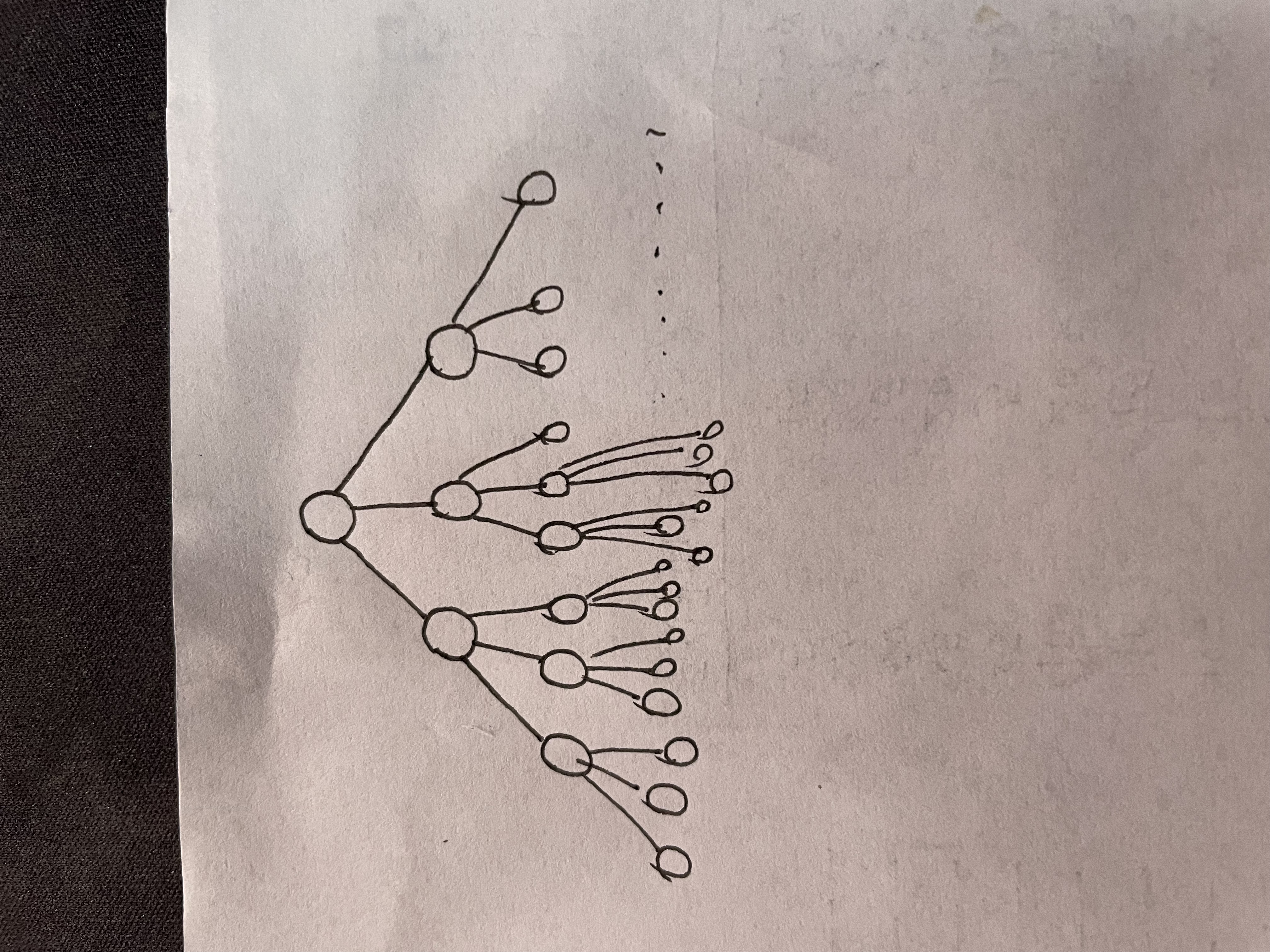# 算法第五章实验报告

7-2 最小重量机器设计问题 (25 分)

### 输入样例:

3 3 4
1 2 3
3 2 1
2 2 2
1 2 3
3 2 1
2 2 2

### 输出样例:

4
1 3 1 1.2解空间树#include <iostream>
using namespace std;

int n;
int m;
int d;
int w;
int c;
int minw=100000000;
int curw=0;
int curc=0;
int minx;
int x;

void backtrack(int t){
if(t>n){
if(curw<minw){
minw=curw;
for(int i=1; i<=n; i++){
minx[i]=x[i];
}
}
}
else{
for(int i=1; i<=m; i++){
curc+=c[t][i];
curw+=w[t][i];
x[t]=i;

if(curc<=d && curw<minw){
backtrack(t+1);
}

curc-=c[t][i];
curw-=w[t][i];
x[t]=0;
}
}
}

int main(){
cin>>n>>m>>d;

for(int i=1; i<=n; i++){
for(int j=1; j<=m; j++){
cin>>c[i][j];
}
}

for(int i=1; i<=n; i++){
for(int j=1; j<=m; j++){
cin>>w[i][j];
}
}

backtrack(1);
cout<<minw<<endl;
for(int i=1; i<=n; i++){
cout<<minx[i]<<" ";
}
cout<<endl;
return 0;
}


2.0我对回溯算法的理解

 
posted @ 2021-12-17 21:58  Russell-H  阅读(4)  评论(0编辑  收藏  举报